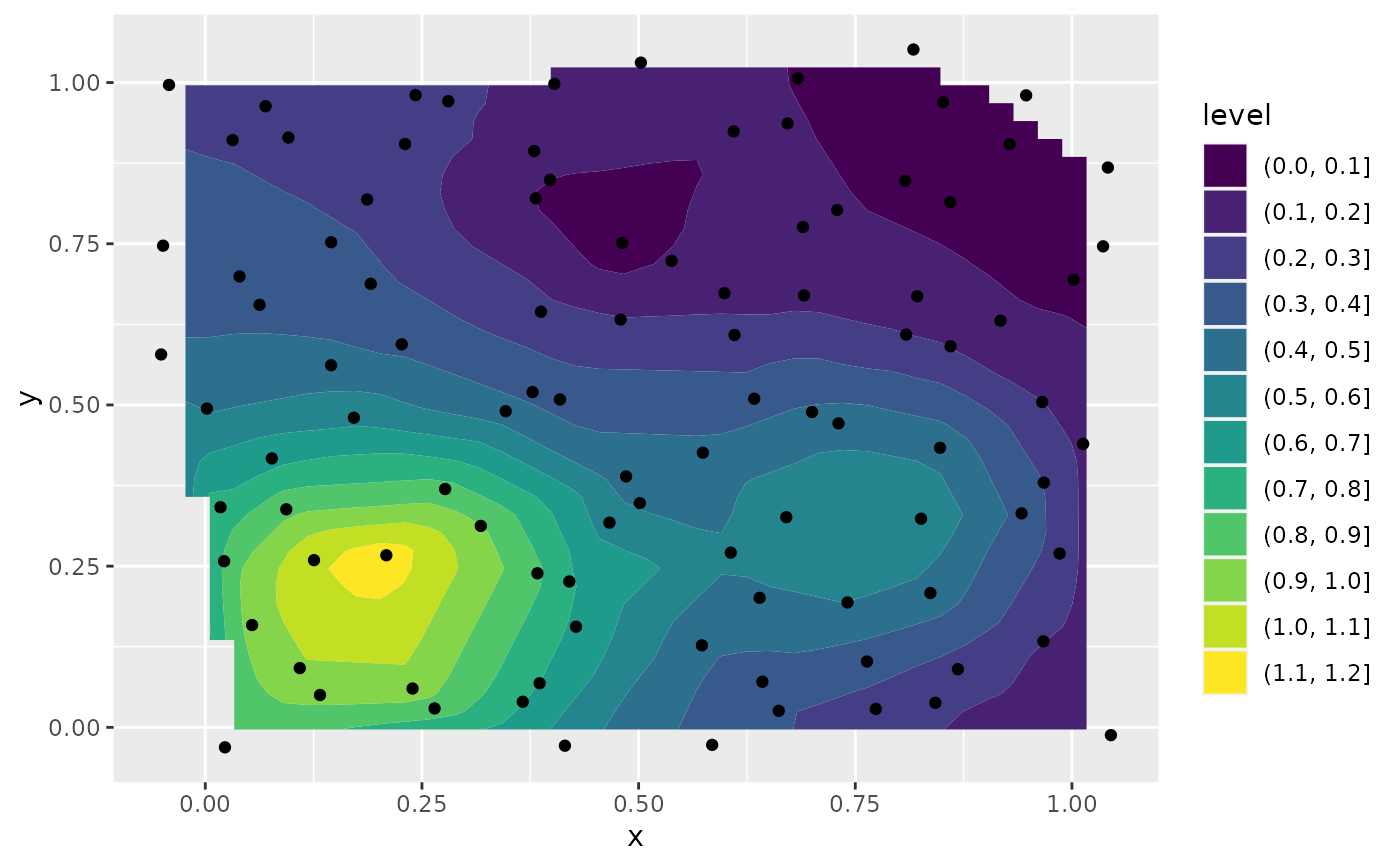ggplot2 can not draw true 3D surfaces, but you can use geom_contour(), geom_contour_filled(), and geom_tile() to visualise 3D surfaces in 2D. To specify a valid surface, the data must contain x, y, and z coordinates, and each unique combination of x and y can appear at most once. Contouring requires that the points can be rearranged so that the z values form a matrix, with rows corresponding to unique x values, and columns corresponding to unique y values. Missing entries are allowed, but contouring will only be done on cells of the grid with all four z values present. If your data is irregular, you can interpolate to a grid before visualising using the interp::interp() function from the interp package (or one of the interpolating functions from the akima package.)

## Usage

geom_contour(
mapping = NULL,
data = NULL,
stat = "contour",
position = "identity",
...,
bins = NULL,
binwidth = NULL,
breaks = NULL,
lineend = "butt",
linejoin = "round",
linemitre = 10,
na.rm = FALSE,
show.legend = NA,
inherit.aes = TRUE
)

geom_contour_filled(
mapping = NULL,
data = NULL,
stat = "contour_filled",
position = "identity",
...,
bins = NULL,
binwidth = NULL,
breaks = NULL,
na.rm = FALSE,
show.legend = NA,
inherit.aes = TRUE
)

stat_contour(
mapping = NULL,
data = NULL,
geom = "contour",
position = "identity",
...,
bins = NULL,
binwidth = NULL,
breaks = NULL,
na.rm = FALSE,
show.legend = NA,
inherit.aes = TRUE
)

stat_contour_filled(
mapping = NULL,
data = NULL,
geom = "contour_filled",
position = "identity",
...,
bins = NULL,
binwidth = NULL,
breaks = NULL,
na.rm = FALSE,
show.legend = NA,
inherit.aes = TRUE
)

## Arguments

mapping

Set of aesthetic mappings created by aes() or aes_(). If specified and inherit.aes = TRUE (the default), it is combined with the default mapping at the top level of the plot. You must supply mapping if there is no plot mapping.

data

The data to be displayed in this layer. There are three options:

If NULL, the default, the data is inherited from the plot data as specified in the call to ggplot().

A data.frame, or other object, will override the plot data. All objects will be fortified to produce a data frame. See fortify() for which variables will be created.

A function will be called with a single argument, the plot data. The return value must be a data.frame, and will be used as the layer data. A function can be created from a formula (e.g. ~ head(.x, 10)).

stat

The statistical transformation to use on the data for this layer, as a string.

position

Position adjustment, either as a string, or the result of a call to a position adjustment function.

...

Other arguments passed on to layer(). These are often aesthetics, used to set an aesthetic to a fixed value, like colour = "red" or size = 3. They may also be parameters to the paired geom/stat.

bins

Number of contour bins. Overridden by binwidth.

binwidth

The width of the contour bins. Overridden by breaks.

breaks

Numeric vector to set the contour breaks. Overrides binwidth and bins. By default, this is a vector of length ten with pretty() breaks.

lineend

Line end style (round, butt, square).

linejoin

Line join style (round, mitre, bevel).

linemitre

Line mitre limit (number greater than 1).

na.rm

If FALSE, the default, missing values are removed with a warning. If TRUE, missing values are silently removed.

show.legend

logical. Should this layer be included in the legends? NA, the default, includes if any aesthetics are mapped. FALSE never includes, and TRUE always includes. It can also be a named logical vector to finely select the aesthetics to display.

inherit.aes

If FALSE, overrides the default aesthetics, rather than combining with them. This is most useful for helper functions that define both data and aesthetics and shouldn't inherit behaviour from the default plot specification, e.g. borders().

geom

The geometric object to use display the data

## Aesthetics

geom_contour() understands the following aesthetics (required aesthetics are in bold):

• x

• y

• alpha

• colour

• group

• linetype

• size

• weight

Learn more about setting these aesthetics in vignette("ggplot2-specs").

geom_contour_filled() understands the following aesthetics (required aesthetics are in bold):

• x

• y

• alpha

• colour

• fill

• group

• linetype

• size

• subgroup

Learn more about setting these aesthetics in vignette("ggplot2-specs").

stat_contour() understands the following aesthetics (required aesthetics are in bold):

• x

• y

• z

• group

• order

Learn more about setting these aesthetics in vignette("ggplot2-specs").

stat_contour_filled() understands the following aesthetics (required aesthetics are in bold):

• x

• y

• z

• fill

• group

• order

Learn more about setting these aesthetics in vignette("ggplot2-specs").

## Computed variables

The computed variables differ somewhat for contour lines (computed by stat_contour()) and contour bands (filled contours, computed by stat_contour_filled()). The variables nlevel and piece are available for both, whereas level_low, level_high, and level_mid are only available for bands. The variable level is a numeric or a factor depending on whether lines or bands are calculated.

level

Height of contour. For contour lines, this is numeric vector that represents bin boundaries. For contour bands, this is an ordered factor that represents bin ranges.

level_low, level_high, level_mid

(contour bands only) Lower and upper bin boundaries for each band, as well the mid point between the boundaries.

nlevel

Height of contour, scaled to maximum of 1.

piece

Contour piece (an integer).

geom_density_2d(): 2d density contours

## Examples

# Basic plot
v <- ggplot(faithfuld, aes(waiting, eruptions, z = density))
v + geom_contour()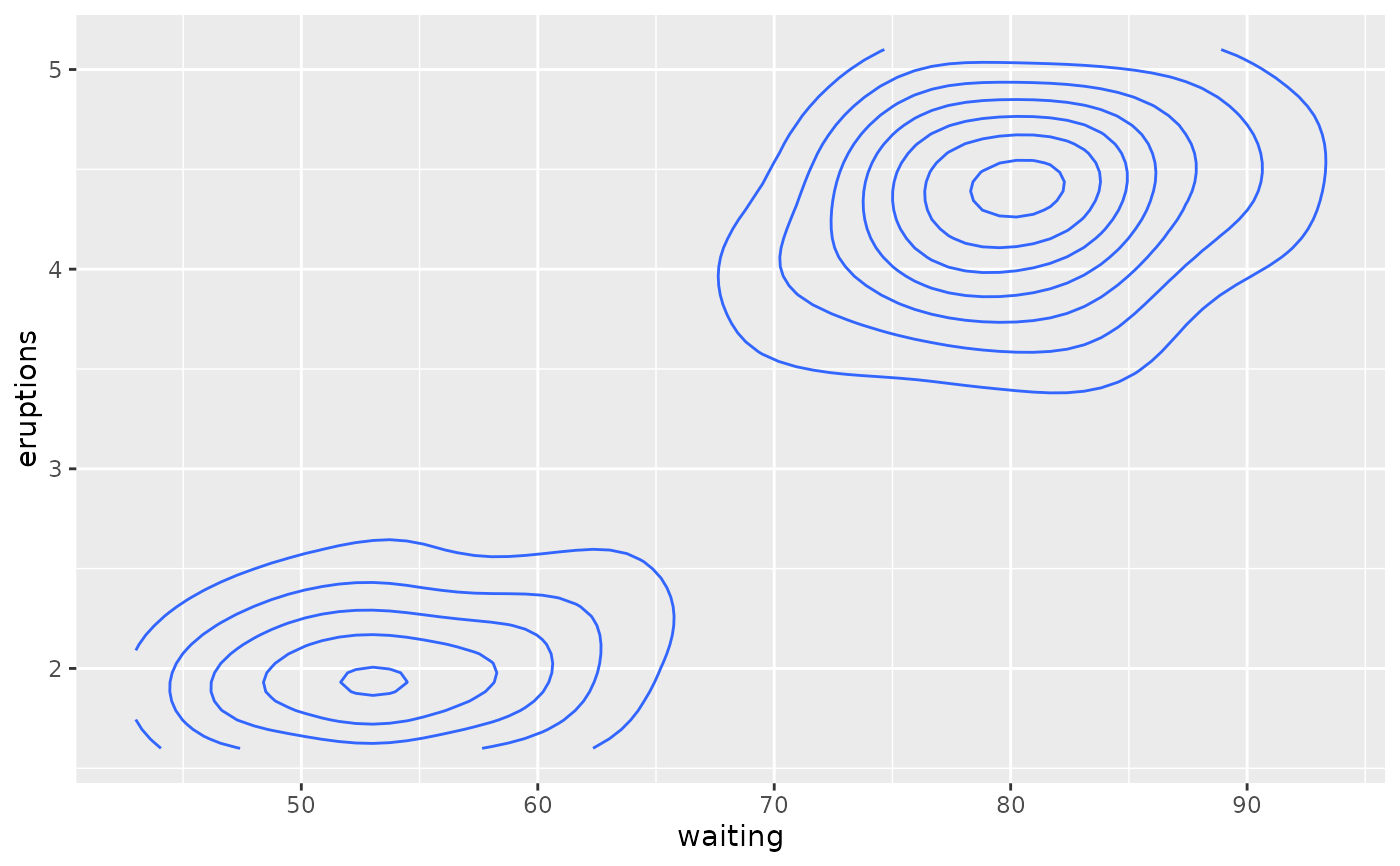# Or compute from raw data
ggplot(faithful, aes(waiting, eruptions)) +
geom_density_2d()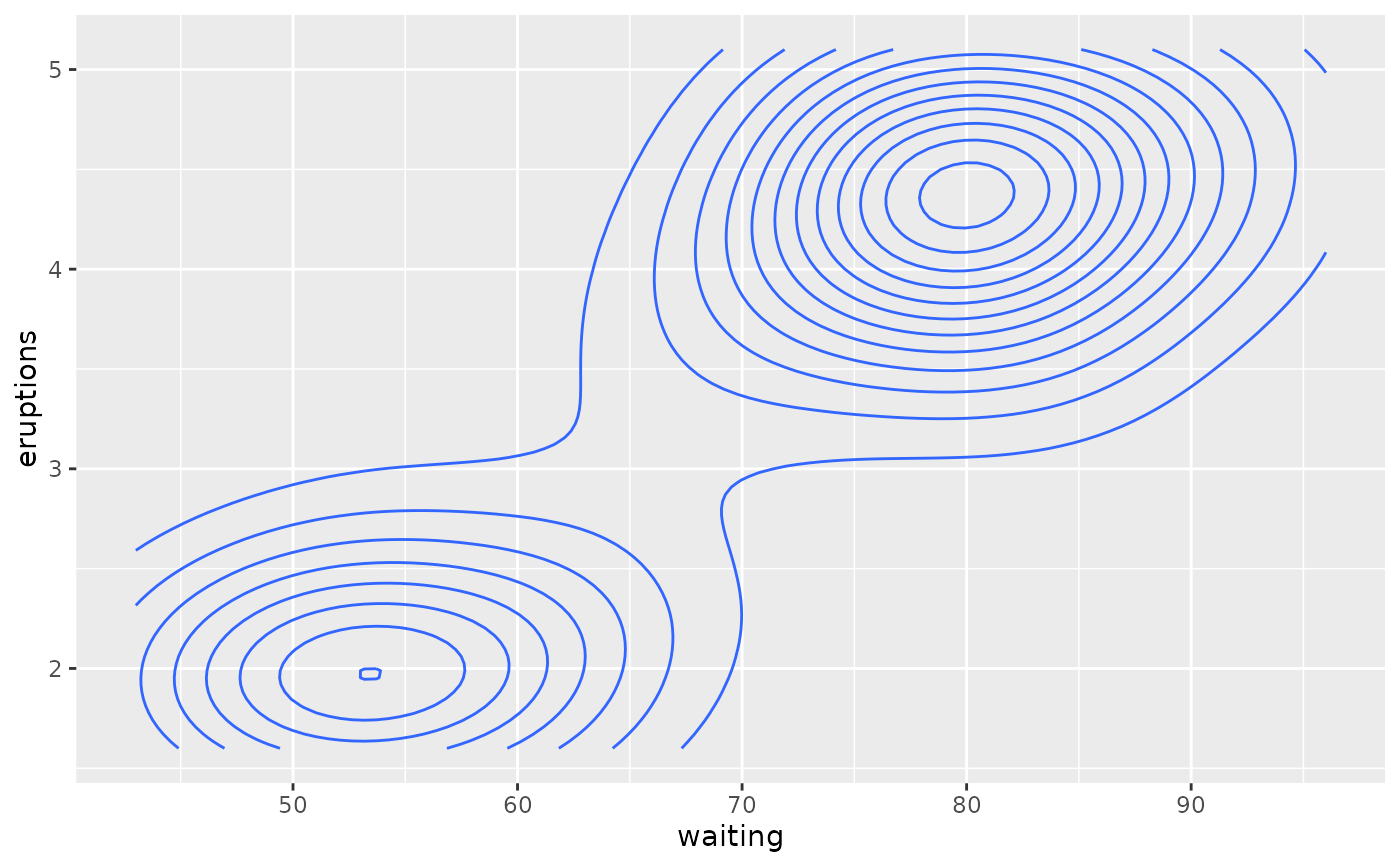# \donttest{
# use geom_contour_filled() for filled contours
v + geom_contour_filled()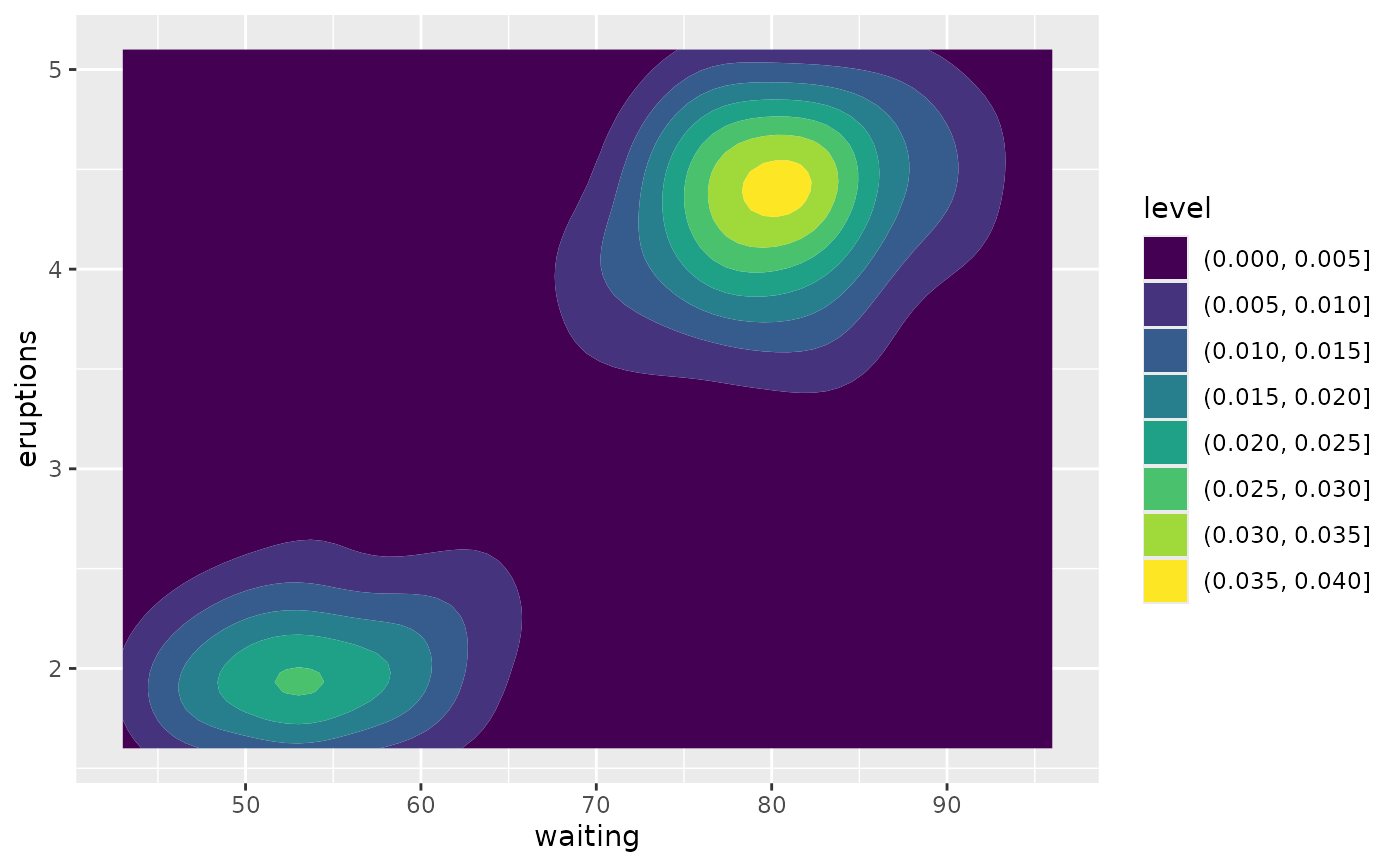# Setting bins creates evenly spaced contours in the range of the data
v + geom_contour(bins = 3)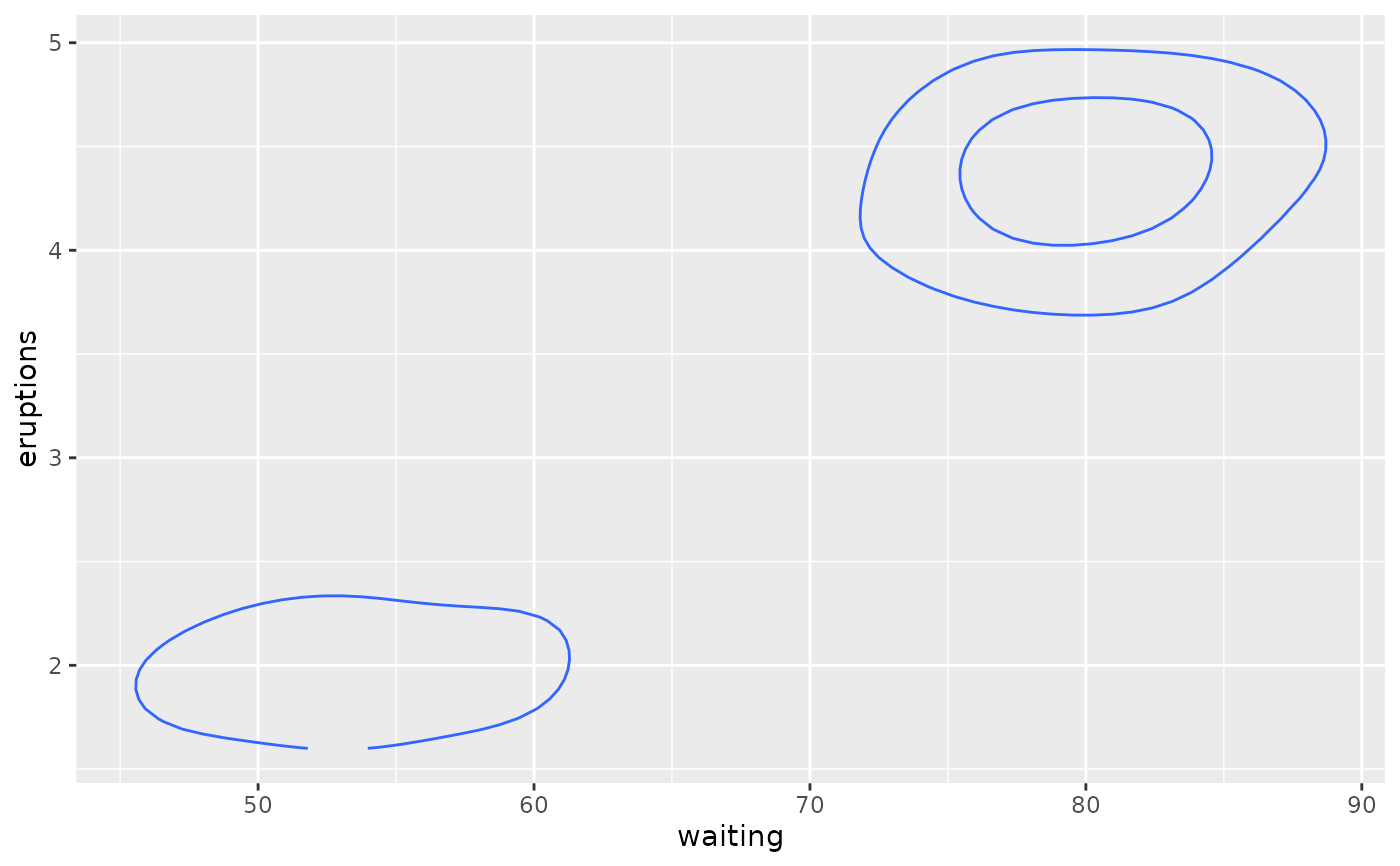v + geom_contour(bins = 5)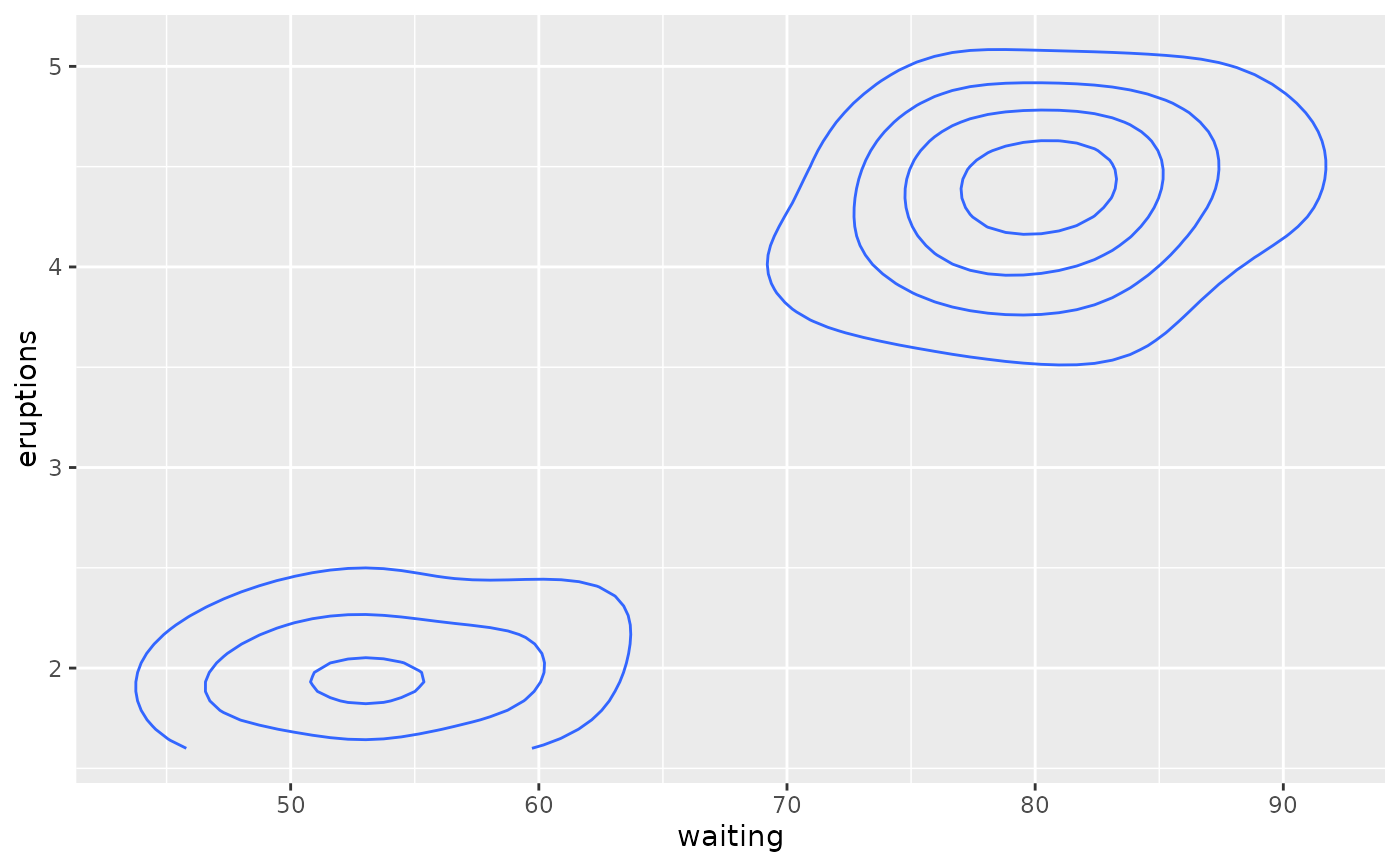# Setting binwidth does the same thing, parameterised by the distance
# between contours
v + geom_contour(binwidth = 0.01)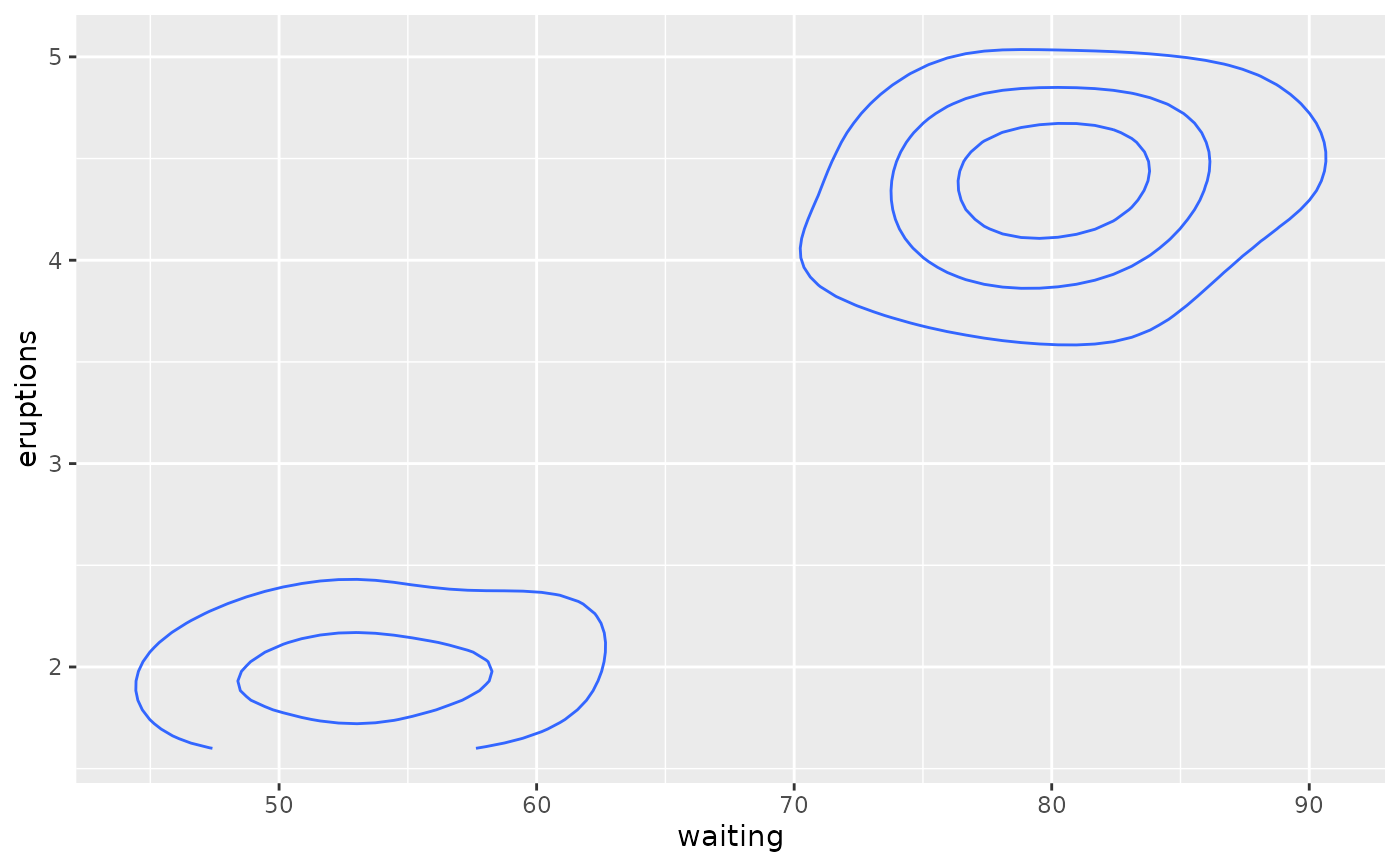v + geom_contour(binwidth = 0.001)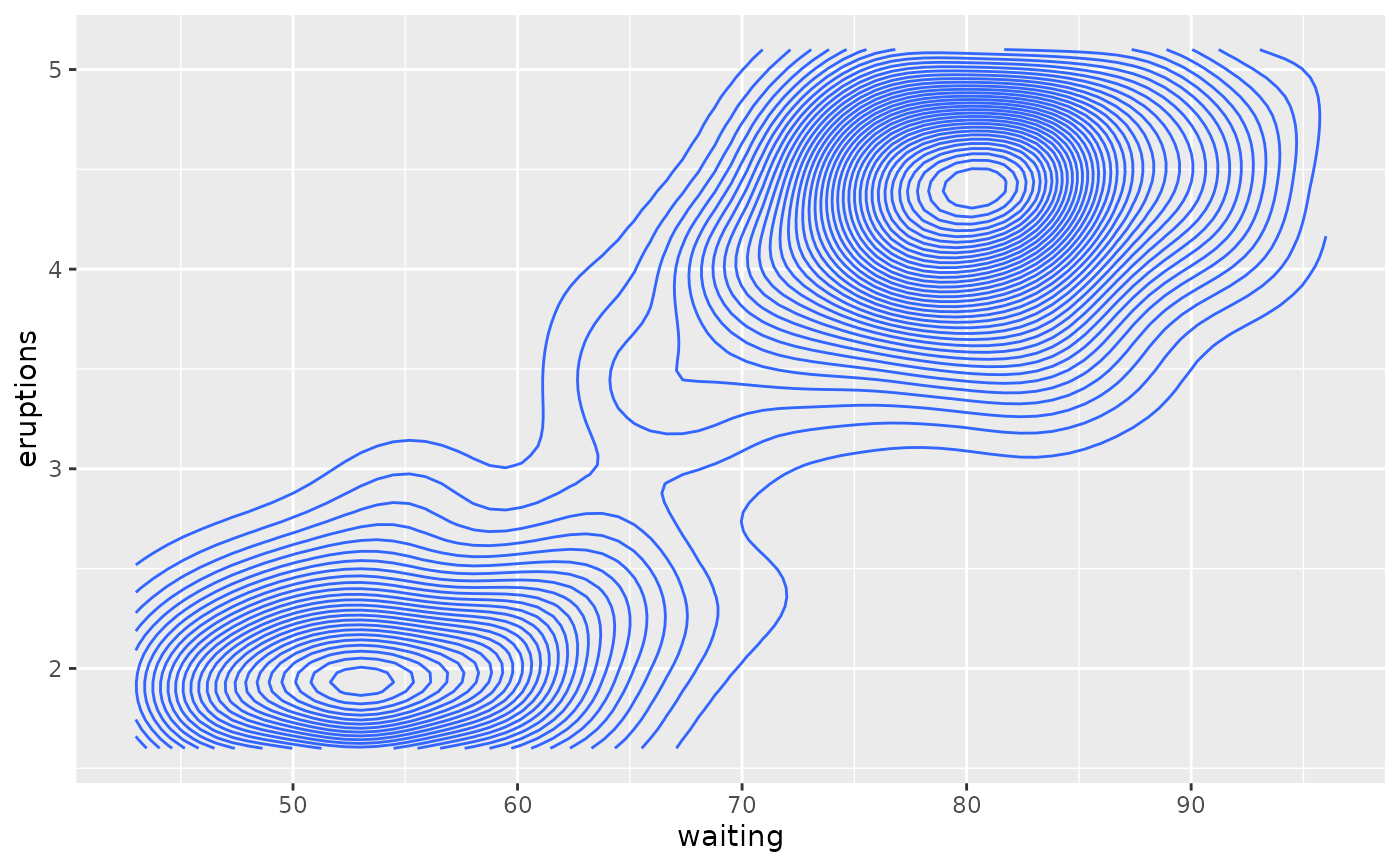# Other parameters
v + geom_contour(aes(colour = after_stat(level)))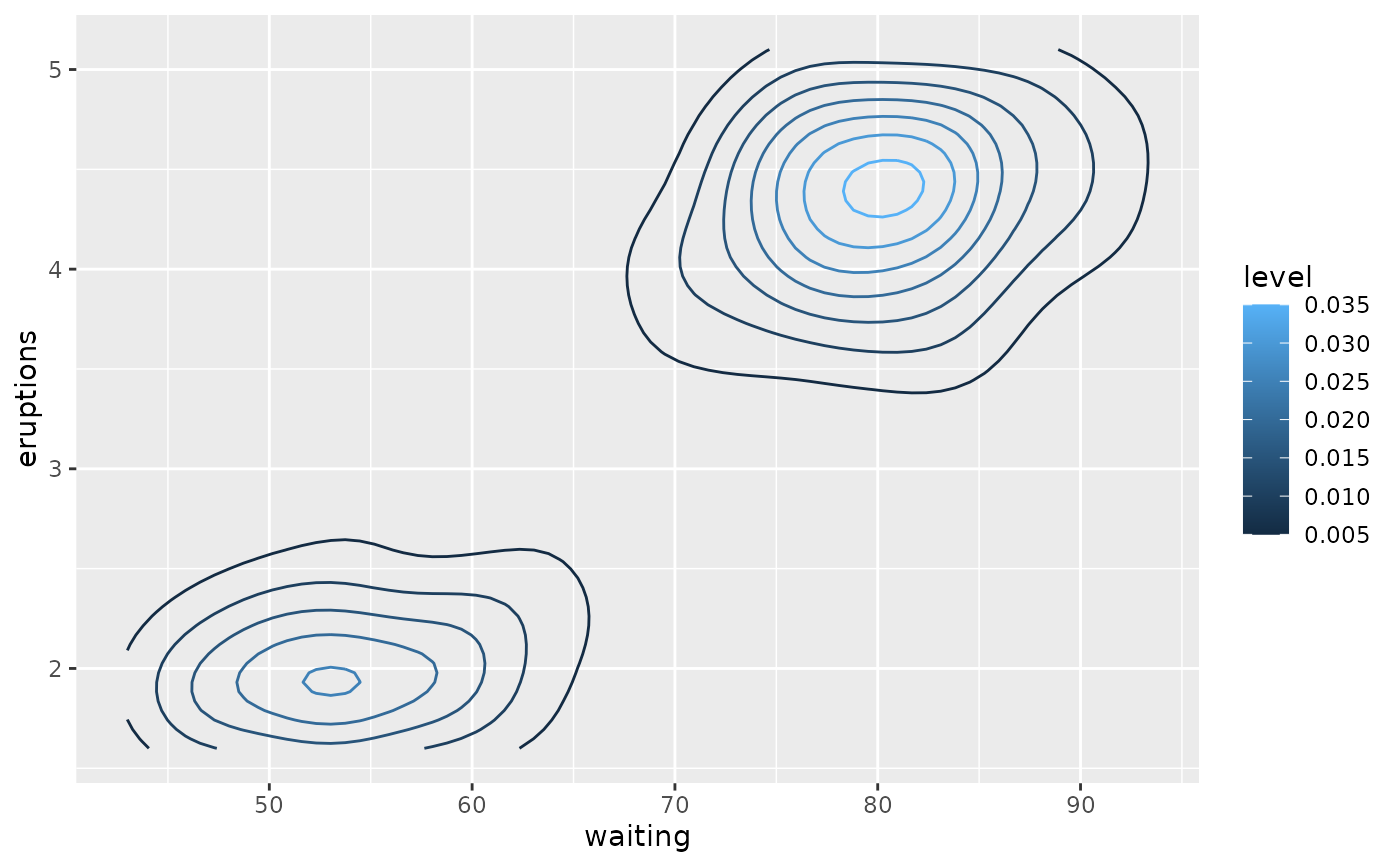v + geom_contour(colour = "red")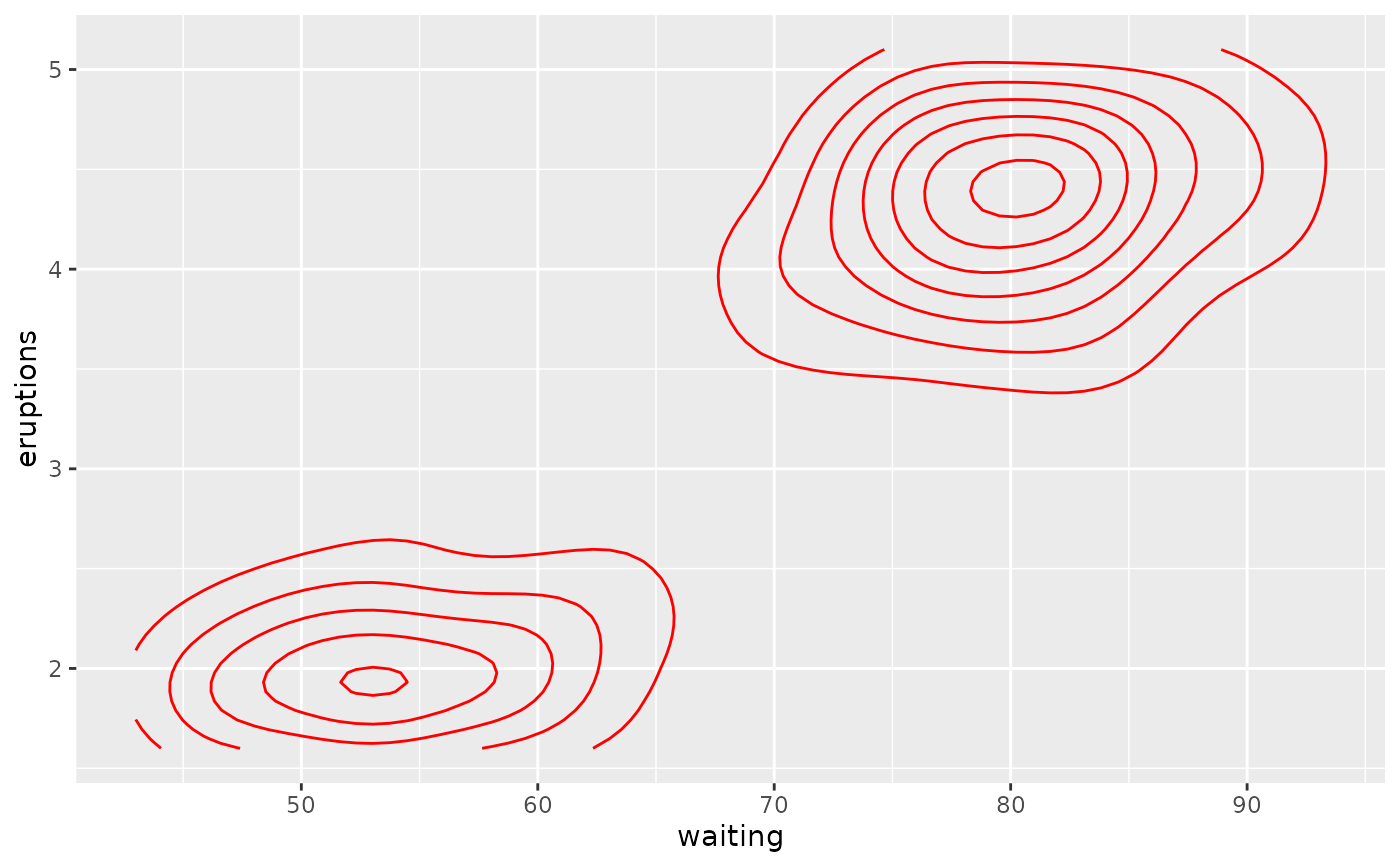v + geom_raster(aes(fill = density)) +
geom_contour(colour = "white")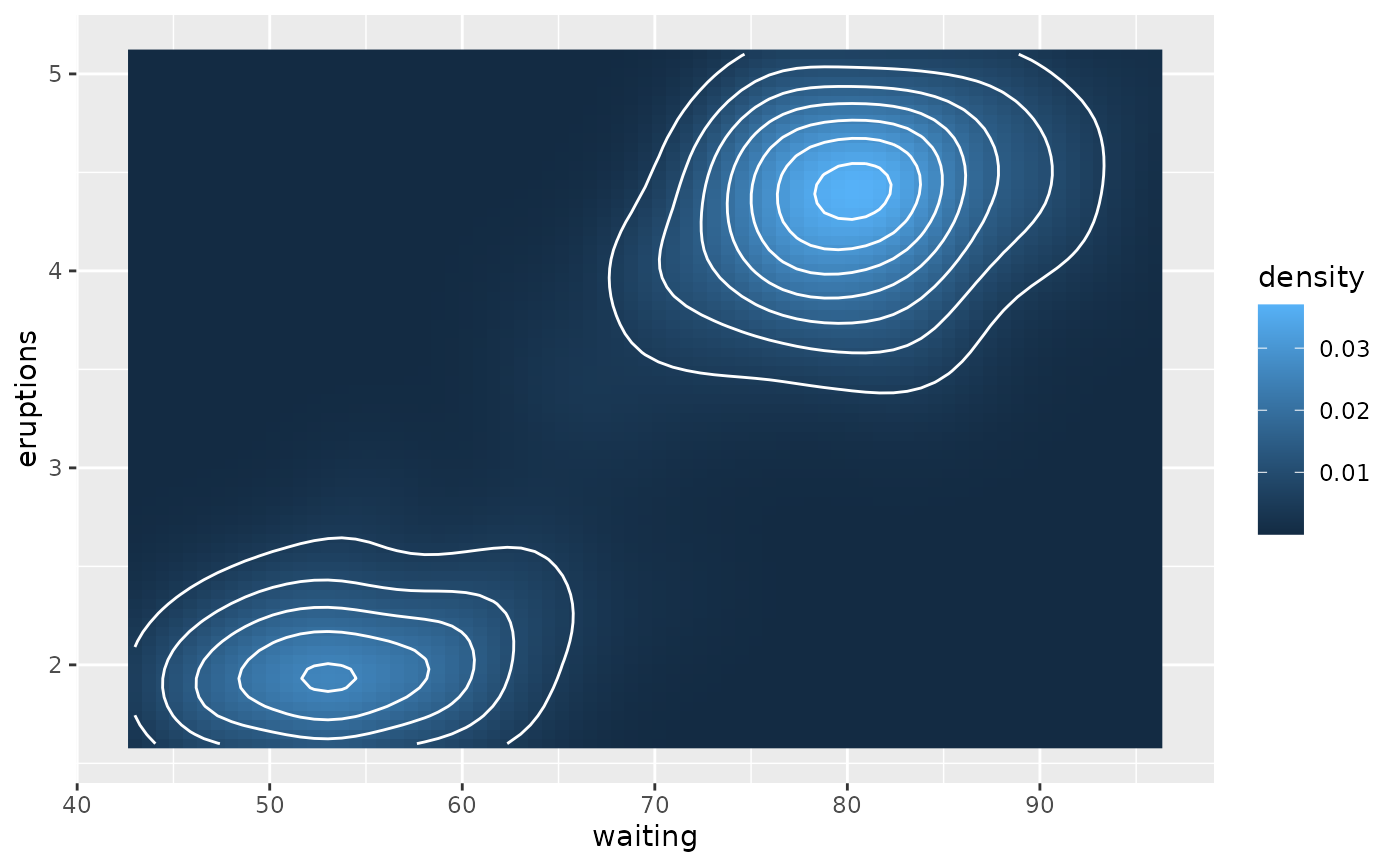# Irregular data
if (requireNamespace("interp")) {
# Use a dataset from the interp package
data(franke, package = "interp")
origdata <- as.data.frame(interp::franke.data(1, 1, franke))
grid <- with(origdata, interp::interp(x, y, z))
griddf <- subset(data.frame(x = rep(grid$x, nrow(grid$z)),
y = rep(grid$y, each = ncol(grid$z)),
z = as.numeric(grid\$z)),
!is.na(z))
ggplot(griddf, aes(x, y, z = z)) +
geom_contour_filled() +
geom_point(data = origdata)
} else
message("Irregular data requires the 'interp' package")